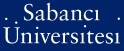# A reflexivity result concerning banach space operators with a multiply connected spectrum

Yavuz, Onur (2010) A reflexivity result concerning banach space operators with a multiply connected spectrum. Integral Equations and Operator Theory, 68 (4). pp. 473-485. ISSN 0378-620X (Print) 1420-8989 (Online)

Full text not available from this repository.

Official URL: http://dx.doi.org/10.1007/s00020-010-1818-3

## Abstract

We consider a multiply connected domain Ω which is obtained by removing n closed disks which are centered at λ j with radius r j for j = 1, . . . , n from the unit disk. We assume that T is a bounded linear operator on a separable reflexive Banach space whose spectrum contains ∂Ω and does not contain the points λ1, λ2, . . . , λ n , and the operators T and r j (T − λ j I)−1 are polynomially bounded. Then either T has a nontrivial hyperinvariant subspace or the WOT-closure of the algebra {f(T) : f is a rational function with poles off $${\overline\Omega}$$} is reflexive.

Item Type: Article Invariant subspaces - reflexive operator algebras - polynomially bounded operators - functional calculus Q Science > QA Mathematics > QA299.6-433 Analysis 16270 Onur Yavuz 03 Jan 2011 16:43 29 Jul 2019 12:05

### Available Versions of this Item

Repository Staff Only: item control page